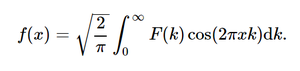sympy.integrals.transforms.inverse_cosine_transform() in Python

• Last Updated : 10 Jul, 2020

With the help of inverse_cosine_transform() method, we can compute the inverse cosine transformation and return the unevaluated function by using this method.inverse cosine function

Syntax : inverse_cosine_transform(F, k, x, **hints)

Return : Return the unevaluated function.

Example #1 :

In this example we can see that by using inverse_cosine_transform() method, we are able to get the inverse cosine transformation and return the unevaluated function.

Python3

 # import inverse_cosine_transformfrom sympy import inverse_cosine_transform, exp, sqrt, pifrom sympy.abc import x, k, a  # Using inverse_cosine_transform() methodgfg = inverse_cosine_transform(sqrt(2)*a/(sqrt(pi)*(a**2 + k**2)), k, x)  print(gfg)

Output :

exp(-a*x)

Example #2 :

Python3

 # import inverse_cosine_transformfrom sympy import inverse_cosine_transform, exp, sqrt, pifrom sympy.abc import x, k, a  # Using inverse_cosine_transform() methodgfg = inverse_cosine_transform(sqrt(2)*a/(sqrt(pi)*(a**2 + k**2)), k, 2)  print(gfg)

Output :

exp(-2*a)

Attention geek! Strengthen your foundations with the Python Programming Foundation Course and learn the basics.

To begin with, your interview preparations Enhance your Data Structures concepts with the Python DS Course. And to begin with your Machine Learning Journey, join the Machine Learning - Basic Level Course

My Personal Notes arrow_drop_up